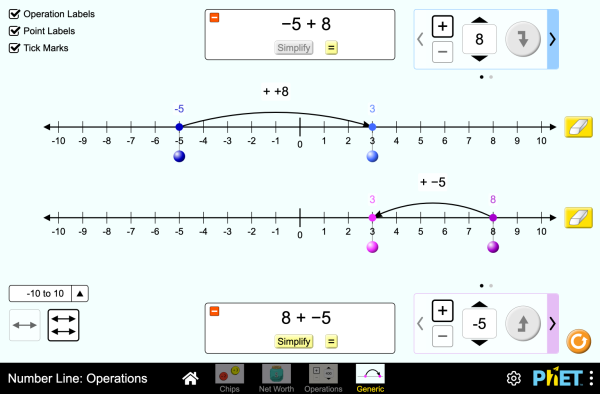# Számegyenes - műveletekLetöltésBeágyazás bezárás A szimuláció futó példányának beágyazása Ezzel a HTML kóddal a szimuláció futó példányát ágyazhatja be weboldalába. A beágyazott szimuláció szélességét, ill. magasságát a "width", ill. a "height" paraméter értékének átállításával szabályozhatja. Olyan kép beágyazása a weboldalba, amelyre kattintva elindul a szimuláció
Kattintásra indul
Ezzel a HTML kóddal egy olyan képernyőfelvételt ágyazhat be weboldalába a szimulációról, melyen a "Kattintásra indul" szöveg olvasható. Operations Számegyenes Integers A PhET egyik támogatójavalamint az Önhöz hasonló oktatók.

• Operations
• Számegyenes
• Integers

### Leírás

Explore operations with integers using an authentic context of net worth, then generalize with a number line. Experiment with adding and subtracting both positives and negatives, and make predictions about whether the total will be positive or negative.

### Lehetséges tanulási célok

• Represent addition and subtraction of integers on a horizontal number line
• Reason about addition and subtraction of integers in terms of number-locations
• Use logical necessity to reason that addition has the opposite effect as subtraction or that adding (or subtracting) a negative integer has the opposite effect as adding (or subtracting) a positive integer.
• Recognize and generate equivalence classes of integer sums and differences
• Show that a number and its additive inverse (opposite) have a sum of 0
• Using a net worth context, describe situations that have a positive sum or difference, negative sum or difference, and zero sum or difference
• View adding a negative as equivalent to subtracting a positive, and explain why this relationship makes sense in contexts such as net worth.
• View subtracting a negative as equivalent to adding a positive, and explain why this relationship makes sense in contexts such as net worth.
• Apply a number line model for addition and subtraction to new contexts.

### Standards Alignment

#### Common Core - Math

7.NS.A.1
Apply and extend previous understandings of addition and subtraction to add and subtract rational numbers; represent addition and subtraction on a horizontal or vertical number line diagram.
7.NS.A.1a
Describe situations in which opposite quantities combine to make 0. For example, a hydrogen atom has 0 charge because its two constituents are oppositely charged.
7.NS.A.1b
Understand p + q as the number located a distance |q| from p, in the positive or negative direction depending on whether q is positive or negative. Show that a number and its opposite have a sum of 0 (are additive inverses). Interpret sums of rational numbers by describing real-world contexts.
7.NS.A.1c
Understand subtraction of rational numbers as adding the additive inverse, p - q = p + (-q). Show that the distance between two rational numbers on the number line is the absolute value of their difference, and apply this principle in real-world contexts.
7.NS.A.1d
Apply properties of operations as strategies to add and subtract rational numbers.
Verzió 1.0.1

### Tanári tippekA szimvezérlők, a modellben foglalt leegyszerűsítések és a hallgatói gondolkodás áttekintése ( PDF ).

### Tanárok által megosztott aktivitások

Integer Addition and Subtraction Assessment Gillian Walker Kp.em.
Kp.al.
Remote
Lab
Hf
Matematika
Nyelv (magyarul) Letöltés vagy Futtatás Tips
angol All EnglishNumber Line: Operations
baszk All EuskaraZenbaki-lerroa: eragiketak
bosnyák All BosanskiBrojevna prava: Operacije
dán All DanskTallinje: Beregninger
francia All françaisDroite numérique: opérations
görög All ΕλληνικάΑριθμογραμμή: πράξεις
holland All NederlandsGetallijn: Bewerkingen
horvát All hrvatskiBrojevna crta: Računske operacije
kínai (hagyományos) All 中文 (台灣)Number Line: Operations_數線：數線的運算
lengyel All polskiOś liczbowa: działania
lett All LatviešuSkaitļu ass: Darbības
maori All MaoriRārangi Tau: Paheko
német All DeutschZahlenstrahl: Operationen
olasz All italianoRetta dei numeri: operazioni
portugál (Brazília) All português (Brasil)Reta Numérica: Operações
spanyol All españolRecta Numérica: Operacionesspanyol (Peru) All español (Perú)Recta numérica: Operaciones
szerb All СрпскиБројевна права: Операције
szlovák All SlovenčinaČíselná os: Operácie
török All TürkçeSayı Doğrusu: İşlemler
vietnami All Tiếng ViệtĐường thẳng số: Các phép toán

HTML5 sims can run on iPads and Chromebooks, as well as PC, Mac, and Linux systems on modern web browsers. If you are experiencing issues using an HTML5 sim on a supported platform, please disable any browser extensions.

iOS 12+ Safari

Android:
Not officially supported. If you are using the HTML5 sims on Android, we recommend using the latest version of Google Chrome.

Chromebook:
The HTML5 and Flash PhET sims are supported on all Chromebooks.
Chromebook compatible sims

Windows Systems: